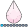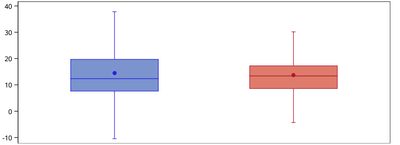## box plot with whiskers to 1.5 IQR.

Hi ,

I have created the following plot.the code I have used is below:

proc sgplot data=&G_DDDATASETNAME noautolegend;
vbox meandos / group=cnsr groupdisplay=cluster
lineattrs=(pattern=1) whiskerattrs=(pattern=solid) meanattrs=(symbol=circlefilled) nooutliers medianattrs=(pattern=2) extreme ;
xaxis ;
yaxis values=(0 to 50 by 1) label ="label for Y axis";
format cnsr mace.;
keylegend / location=outside position=bottom across=1 title="";
run;

Now the issue im facing is to draw the whiskers to 1.5IQR. How do I achieve it?

Thanks

1 ACCEPTED SOLUTION

Accepted SolutionsDanH_sas
SAS Super FREQ

## Re: box plot with whiskers to 1.5 IQR.

Instead of using a HIGHLOW plot for the whiskers, use a SCATTER plot with Y error bars. Then, you can use CAPSCALE to control the size of the caps.

23 REPLIES 23

## Re: box plot with whiskers to 1.5 IQR.

Data might be nice.

Do you mean that you need a "whisker" that goes to 1.5 * IQR when there is actually not data in your data set that far out? By default the whiskers will go to 1.5 times the IQR (plus/minus) but caps at the extreme value encountered if there aren't outliers.

## Re: box plot with whiskers to 1.5 IQR.

I have calculated the 1.5*IQR which doesnt match with this Whiskers.

I see the in the below link that "The whiskers are drawn from the box to the most extreme point that is less than or equal to 1.5 times the IQR". So the Whisker length is not always uto 1.5 IQR is what I understand.

https://documentation.sas.com/?cdcId=pgmsascdc&cdcVersion=9.4_3.5&docsetId=grstatproc&docsetTarget=n...DanH_sas
SAS Super FREQ

## Re: box plot with whiskers to 1.5 IQR.

If you have computed the 1.5IQR, you can use that information to set an appropriate whisker value using the WHISKERPCT option.

Hope this helps!

Dan

## Re: box plot with whiskers to 1.5 IQR.

Hello DanH_sas
May I know how? I see we can give the whiskerpct as 0,10,25 only .DanH_sas
SAS Super FREQ

## Re: box plot with whiskers to 1.5 IQR.

You can specify any value between 0 to 25. Here is the doc for the option:

https://go.documentation.sas.com/?cdcId=pgmsascdc&cdcVersion=9.4_3.5&docsetId=grstatproc&docsetTarge...

## Re: box plot with whiskers to 1.5 IQR.

Hi,

I see the doc sates whiskerpct . So if I calculate 1.5*IQR(say it is whis_iqr) , then can I give as whsketpct=whis_iqr ?djrisks
Barite | Level 11

## Re: box plot with whiskers to 1.5 IQR.

Okay, that's interesting. So are the Whiskers that are produced my SAS smaller than yours? Perhaps, you could look into the WHISKERPCT option.

A definite solution, is that because you have already calculated the necessary statistics, you could look into using GTL and BOXPLOTPARM statement.

## Re: box plot with whiskers to 1.5 IQR.

@Manj wrote:
I have calculated the 1.5*IQR which doesnt match with this Whiskers.

I see the in the below link that "The whiskers are drawn from the box to the most extreme point that is less than or equal to 1.5 times the IQR". So the Whisker length is not always uto 1.5 IQR is what I understand.

https://documentation.sas.com/?cdcId=pgmsascdc&cdcVersion=9.4_3.5&docsetId=grstatproc&docsetTarget=n...

So the answer to my question is: Yes, I want to draw line further out than the data actually exists within the 1.5 IQR.

The first time I was introduced to Box-and-whisker plots the rule was the whiskers indicate the data, not the calculation. The purpose of a box-and-whisker is to show distribution of data in a concise form. If you draw a whisker further then you are implying that there is data there. Hence, fabricating data.djrisks
Barite | Level 11

## Re: box plot with whiskers to 1.5 IQR.

Hi @Manj ,

I believe if you remove the extreme and nooutlier options from your your VBOX plot statement, then you will get the boxplot that you want.

Thanks,

Kriss

## Re: box plot with whiskers to 1.5 IQR.

This isnt helping.djrisks
Barite | Level 11

## Re: box plot with whiskers to 1.5 IQR.

As @ballardw mentioned, the Whiskers will go to 1.5 * the IQR range by default. I'm not sure why this isn't working, and it's difficult to diagnose without the data and/or plot/

## Re: box plot with whiskers to 1.5 IQR.

@Manj wrote:
This isnt helping.

What "isn't helping"?

Data.

Data is good.

If you don't want to provide any then use an existing SAS supplied data set. Such as

```proc sgplot data=sashelp.class noautolegend;
vbox height / group=sex groupdisplay=cluster;
run;```

So, show us using the SASHELP.CLASS data, 1) how you calculated the 1.5 IQR and 2) what value(s) you want displayed on the graph for as generated above.

I will say if I am shown a graph with a whisker that does not have corresponding data in the source that I would be very upset when I found out.

## Re: box plot with whiskers to 1.5 IQR.

Hi ,

I have calculated the IQR as p75-p25

so the whisker ends will be p75+1.5 IQR and p25-1.5 IQR. But it doesnt seems to match with plots whisker ends.

I tried with the following code:

```proc sgplot data=sashelp.class noautolegend;
vbox height / group=sex groupdisplay=cluster;
run;```

## Re: box plot with whiskers to 1.5 IQR.

Hello ,

I have re-written my code as below to generate the boxplot. The problem i face now is (1) how to make the horizontal line within the box to dotted line? (2) how to increase the length of the 'caps' for the lines?

proc sgplot data=dddata.aaa nocycleattrs;

highlow x=cnsr high=iqr_plus low=p75 / group=cnsrtxt groupdisplay=cluster
clusterwidth=0.7 highcap=serif lineattrs=(pattern=1);

highlow x=cnsr high=p75 low=median / group=cnsrtxt type=bar
groupdisplay=cluster grouporder=ascending clusterwidth=0.7
barwidth=0.7 name='a';

highlow x=cnsr high=median low=p25 / group=cnsrtxt type=bar
groupdisplay=cluster grouporder=ascending clusterwidth=0.7
barwidth=0.7 ;

highlow x=cnsr high=p25 low=iqr_neg / group=cnsrtxt groupdisplay=cluster
clusterwidth=0.7 lowcap=serif lineattrs=(pattern=1);

scatter x=cnsr y=mean / group=cnsrtxt groupdisplay=cluster
grouporder=ascending clusterwidth=0.7 markerattrs=(size=9 symbol=circlefilled );

keylegend 'a' / location=outside position=bottom across=1 title="";

xaxis display=none;

run;Discussion stats
• 23 replies
• 1515 views
• 3 likes
• 4 in conversation